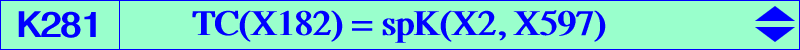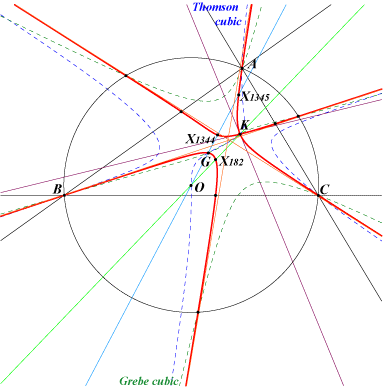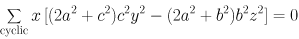X(2), X(6), X(182), X(996), X(1001), X(1344), X(1345), X(4846), X(5967), X(10002), X(14609), X(18775), X(46023), X(46024) X(46023), X(46024) = isogonal conjugates of X(1340), X(1341) points at infinity of the Thomson cubic vertices of the Grebe triangleK281 is a nodal cubic with node K, the nodal tangents being parallel to the asymptotes of the circum-conic through G and K. It has three real asymptotes parallel to those of the Thomson cubic. The tangents at the vertices of the Grebe triangle G1G2G3 concur at the intersection X(14968) of the lines X(2)X(32) and X(6)X(528). See also Table 57. Locus properties : locus of M such that the lines KM and GM* are parallel (M* isogonal conjugate of M). See CL055. locus of M such that the lines GM and KM* intersect on the circumcircle. locus of M such that the trilinear polar of M is perpendicular to the line MX(182). See CL040 for a generalization. see Q012, property 3 for another generalization. The isogonal transform of K281 is K280. These two cubics generate a pencil containing K018 (the second Brocard cubic) and three pK which are K282, K283, K284.